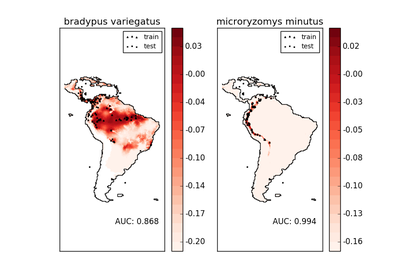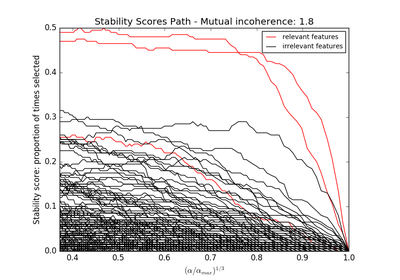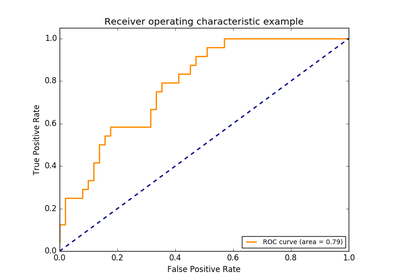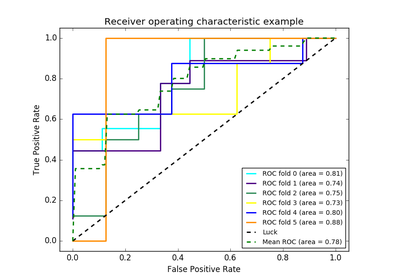# `sklearn.metrics`.auc¶

`sklearn.metrics.``auc`(x, y, reorder=False)[source]

Compute Area Under the Curve (AUC) using the trapezoidal rule

This is a general function, given points on a curve. For computing the area under the ROC-curve, see `roc_auc_score`.

Parameters: x : array, shape = [n] x coordinates. y : array, shape = [n] y coordinates. reorder : boolean, optional (default=False) If True, assume that the curve is ascending in the case of ties, as for an ROC curve. If the curve is non-ascending, the result will be wrong. auc : float

`roc_auc_score`
Computes the area under the ROC curve
`precision_recall_curve`
Compute precision-recall pairs for different probability thresholds

Examples

```>>> import numpy as np
>>> from sklearn import metrics
>>> y = np.array([1, 1, 2, 2])
>>> pred = np.array([0.1, 0.4, 0.35, 0.8])
>>> fpr, tpr, thresholds = metrics.roc_curve(y, pred, pos_label=2)
>>> metrics.auc(fpr, tpr)
0.75
```

## Examples using `sklearn.metrics.auc`¶Species distribution modelingSparse recovery: feature selection for sparse linear models